# NCERT Solutions for Class 5 Maths Can you See The Pattern## myCBSEguide App

CBSE, NCERT, JEE Main, NEET-UG, NDA, Exam Papers, Question Bank, NCERT Solutions, Exemplars, Revision Notes, Free Videos, MCQ Tests & more.

NCERT solutions for Class 5 Mathematics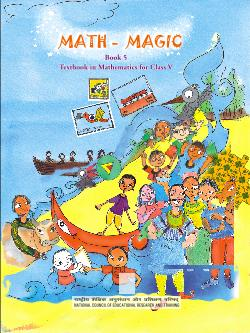## NCERT Solutions for Class 5 Maths Can you See The Pattern

CHAPTER-7

CAN YOU SEE THE PATTERN

###### 1. What should come next?

(a)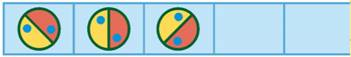(b)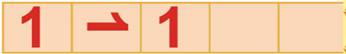(c)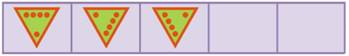(d)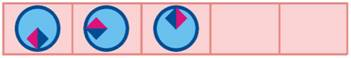Ans. (a)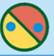____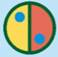____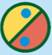____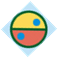____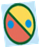(b)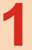____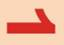____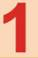____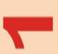____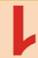(c)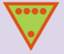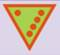____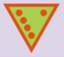________(d)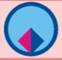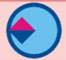____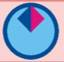____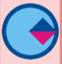____###### 2. See this pattern

____(a)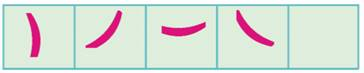The rule of the pattern is — turning by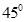each time. Which will be the next? Tick the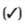right one.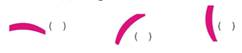Ans.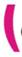###### 3. Magic Squares

____Do you remember magic triangles? Cone now, let’s make some magic squares.

Q. Fill this square using all the numbers from 46 to 54.

Ans. In this magic square, the sum of each of the row of numbers (across down and diagonally) is always the same. We have to complete the magic squares, remembering that the numbers in each line are equal to 150.

Clearly:

In 3 rd row: The required number= 150-52-47=150-99=51

In 3 rd column: The required number =150-49-47=150-96=54

In 2 nd row: The required number = 150-46-54= 150-100=50

In 2 nd column: The required number= 150-50-52= 150-102=188

In 1 st row: The required number= 150-18-49=150-97= 53

Therefore, the complete magic square is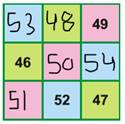###### Q. Fill this square suing all the numbers from 21 to 29.

Rule: The total of each side is 75.

Ans. Let us fix 26 on the top most left hand side box.

Taking the diagonal of the square, we have

26+25=51 and 75-51=24

Therefore, put 24 at the end of this diagonal.

Fix 22 on the top most-right side box.

Taking the diagonal in which 22 lies, we have

22+25=47 and 75-47=28

Therefore, put 28 at the end of this diagonal.

Clearly,

In 1 st row: The required number =75-(26+22) =75-48=27

In 1 st column: The required number = 75-(26+28) =75-54=21

In 2 nd row: The required number =75- (21+25) =75-46=29

In 2 nd column: The required number =75-(28+24) =75-52=27

Therefore, the complete magic square is as shown below: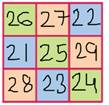###### 3. Fill in the blank spaces in the same way.

(a) 14+…..+……=34+24+20

(b) ……+ 42+ ……=65+…. +80

(c) 200 + 300 + ….. = ….. + 400 + …….

(d) ….. + ….. + ….. = ….. + ….. + …….

Ans. (a) 14 + 20 + 34 = 34 + 14 +20

(b) 80 + 42 + 65 = 65 + 42 + 80

(c) 200 + 300 + 400 = 300 + 400 + 200

(d) 34 + 29 +47 = 47 + 34 +29

###### 4. Now you try and change these numbers into special numbers:

(a) 28 (b) 132 (c) 273

Ans. (a)

 Given number 28 Then turn it back to front 82 Then add them two togetherIt is not a special number Now carry on with the number 110 Again turn it back two together 011 Then add them two together 121 121 is the required special number

(b)

 Given number 132 Then turn it back to front 231 Then add them two together 363 363 is the required special number

____

(c)

 Given number 273 Then turn it back to front 372 Then add them two together 645 It is not a special number Now carry on with the number 645 Again turn it back two together 546 Then add them two together 1191 It is not a special number Now carry on with the number 1191 Again turn it back two together 1911 Then add them two together 3102 It is not a special number Now carry on with the number 3102 Again turn it back two together 2013 Then add them two together 5115 5115 is the required special number

NCERT Solutions for Class 5 Maths Can you See The Pattern

###### 5. Choose any 3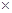3 box from a calendar and find the total in the same way. Play this game with your family.

Ans.Let us mark a 33 box (9 dates) on the calendar and see some magic.

 S M T W T F S 1 2 3 4 5 6 7 8 9 10 11 12 13 14 15 16 17 18 19 20 21 22 23 24 25 26 27 28 29 30 31

Take the smallest number: 2

10

Multiply it by 99

Total 90

###### 6. Take any number. Now multiply it by 2, 3, …… at every step. Also add 3 to it at each step. Look at the difference in the answer. Is it the same at every step?

122 + 3 = 27

123 + 3 = 39

124 + 3 = 51

125 + 3 = 63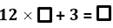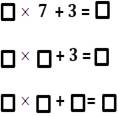Ans. Filling in the blank boxes, we have

126 + 3 = 75

127 + 3 = 87

128 + 3 = 99

129 + 3 = 111

NCERT Solutions for Class 5 Maths Can you See The Pattern

###### 7. Look at the numbers below. Look for the pattern. Can you take it forward?

(9 – 1) ÷ 8 = 1

(98 – 2) ÷ 8 = 12

(987 – 3) ÷ 8 = 123

(9876 – 4) ÷ 8 = ____

(98765 – 5) ÷ 8 = ____

( ____–__ ) ÷ 8 = ____

( ____–__ ) ÷ 8 = ____

Ans. Yes, the given pattern can be taken forward as under:

(9 – 1) ÷ 8 = 1

(98 – 2) ÷ 8 = 12

(987 – 3) ÷ 8 = 123

(9876 – 4) ÷ 8 = 1234

(98765 – 5) ÷ 8 = 12345

(987654 – 6) ÷ 8 = 123456

(9876543 – 7) ÷8 = 1234567

NCERT Solutions for Class 5 Maths Can you See The Pattern

1 +2 +3 +4 +5 +6 +7 +8 +9 +10 = 55

11+12+ .. + ..  + .. + ..   +..  + .. + .. +20 = 155

21+ .. + .. + .. + .. + .. + .. + .. + .. +30 = …

31+ .. + .. + .. + .. + .. + .. + .. + .. +40 = …

41+ .. + .. + .. + .. + .. + .. + .. + .. +50 = …

51+ .. + .. + .. + .. + .. + .. + .. + .. +60 = 555

61+ .. + .. + .. + .. + .. + .. + .. + .. +70 = …

Ans. 1 +2 +3 +4 +5 +6 +7 +8 +9 +10 = 55

11+12+13 +14 +15 +16 +17 +18 +19 +20 = 155

21+22 +23 +24 +25 + 26+27 +28 +29 +30 = 255

31+32 +33 +34 +35 +36 +37 +38 +39+40 = 355

41+42 +43 +44 +45 +46 +47 +48+49 +50 = 455

51+52 +53 +54 +55 +56 +57 +58+59 +60 = 555

61+62+63+64 +65 +66 +67 +68 +69 +70 = 655

NCERT Solutions for Class 5 Maths Can you See The Pattern

###### 9. Take the first two odd numbers, now add the, see what you get.

Now, at every step, add the next odd number.

1 + 3 = 4 = 22

1 + 3 + 5 = 9 = 33

1 + 3 + 5 + 7 = 16 = 44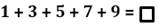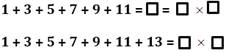How far can you go on?

Ans. Let us complete it.

1 + 3 + 5 + 7 +9 = 25 = 55

1 + 3 + 5 + 7 + 9 + 11 = 36 = 66

1 + 3 + 5 + 7 + 9 + 11 + 13 = 49 = 77

NCERT Solutions for Class 5 Maths Can you See The Pattern

###### Banno and binod were playing a guessing game by writing clues about a secret number. Each tried by writing clues about a secret number. Each tried to guess the other’s secret number from the clues.

Can you guess their secret numbers?

(a) It is larger than half of 100.

Ans. (a) It is larger than half of 100 means > 50.

(b) It is more than 6 tens and less than 7 tens.

Ans. (b) It is more than 6 tens and less than 7 tens it lies between 60 and 70.

(c) The tens digit is one more than he one’s digit.

Ans. (c) The tens digit is one more than one’s digit is 6-5 =5.

(d) Together the digits have a sum of 11.

Ans. (d) Together the digits have the sum of 11, so the number is 65.

NCERT Solutions for Class 5 Maths Can you See The Pattern

###### 11. Write a set of clues for a secret number of your own. Then give it to a friend to guess your secret answer.

Ans. A set of clues to find secret numbers are:

____ It is larger than half of 100.

It is more than 7 tens and less than 8 tens.

The tens digit is one less than the one’s digit.

Together the digits have a sum of 15.

NCERT Solutions for Class 5 Maths Can you See The Pattern

###### 12. (a) Ask your friend-Write down his age. Add 5 to it. Multiply the sum by 2. Subtract 10 from it. Next divide it by 2. What do you get?

Ans.(a) Age: 7

Add 5 to it: 7 + 5 =12

Multiply the sum by 2= 122 =24

Subtract 10 from it = 24 -10 =14

Divide it by 2 =14/2= 7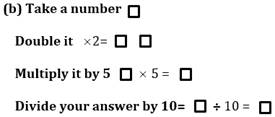Ans. (b) Take a number as 5(say)

Double it 52 = 10

Multiply by 5 = 105 = 50

Thus, we got the supposed answer.

(c) Look at this pattern of number and take it forward.

1 = 11

121 = 1111

12321 = 111111

1234321 = ?

Ans. (c) Taking the pattern forward, we have

1234321 = 11111111.

## NCERT Solutions for Class 5 Mathematics Chapter 7 Can you See The Pattern

NCERT Solutions Class 5 Maths PDF (Download) Free from myCBSEguide app and myCBSEguide website. Ncert solution class 5 Maths includes text book solutions from Class 5 Maths Book . NCERT Solutions for CBSE Class 5 Maths have total 14 chapters. 5 Maths NCERT Solutions in PDF for free Download on our website. Ncert Maths class 5 solutions PDF and Maths ncert class 5 PDF solutions with latest modifications and as per the latest CBSE syllabus are only available in myCBSEguide.

## CBSE app for Students

To download NCERT Solutions for Class 5 Maths, Social Science Computer Science, Home Science, Hindi English, Maths Science do check myCBSEguide app or website. myCBSEguide provides sample papers with solution, test papers for chapter-wise practice, NCERT solutions, NCERT Exemplar solutions, quick revision notes for ready reference, CBSE guess papers and CBSE important question papers. Sample Paper all are made available through the best app for CBSE students and myCBSEguide website.